# 8 20 V1 12 3 2 In the above circuit, V1 is 35 volts, and V2...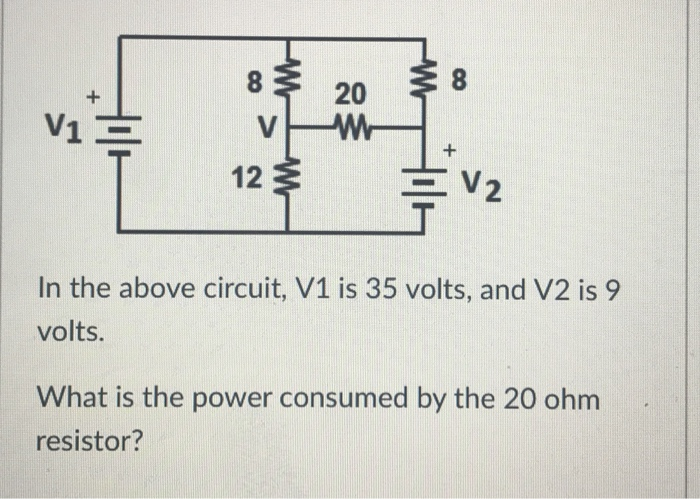8 20 V1 12 3 2 In the above circuit, V1 is 35 volts, and V2 is 9 volts. What is the power consumed by the 20 ohm resistor?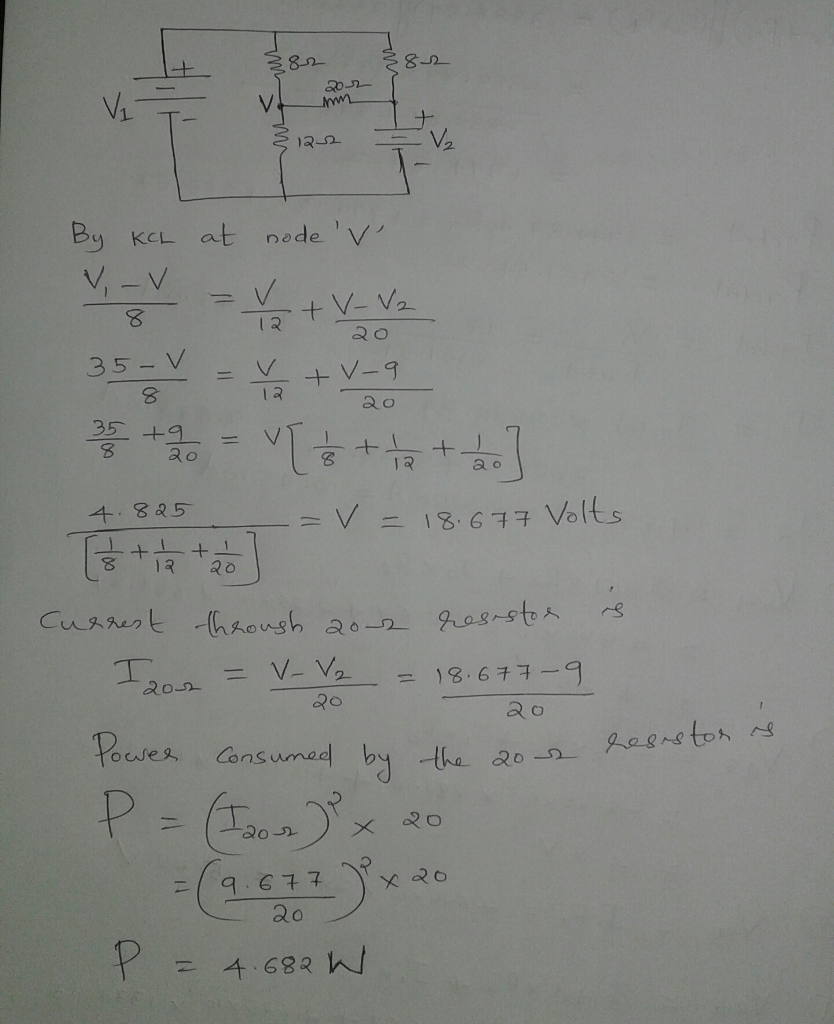#### Earn Coin

Coins can be redeemed for fabulous gifts.

Similar Homework Help Questions
• ### R1 2 In the above circuit, V1- 18 volts and V2 -9 volts. If each of...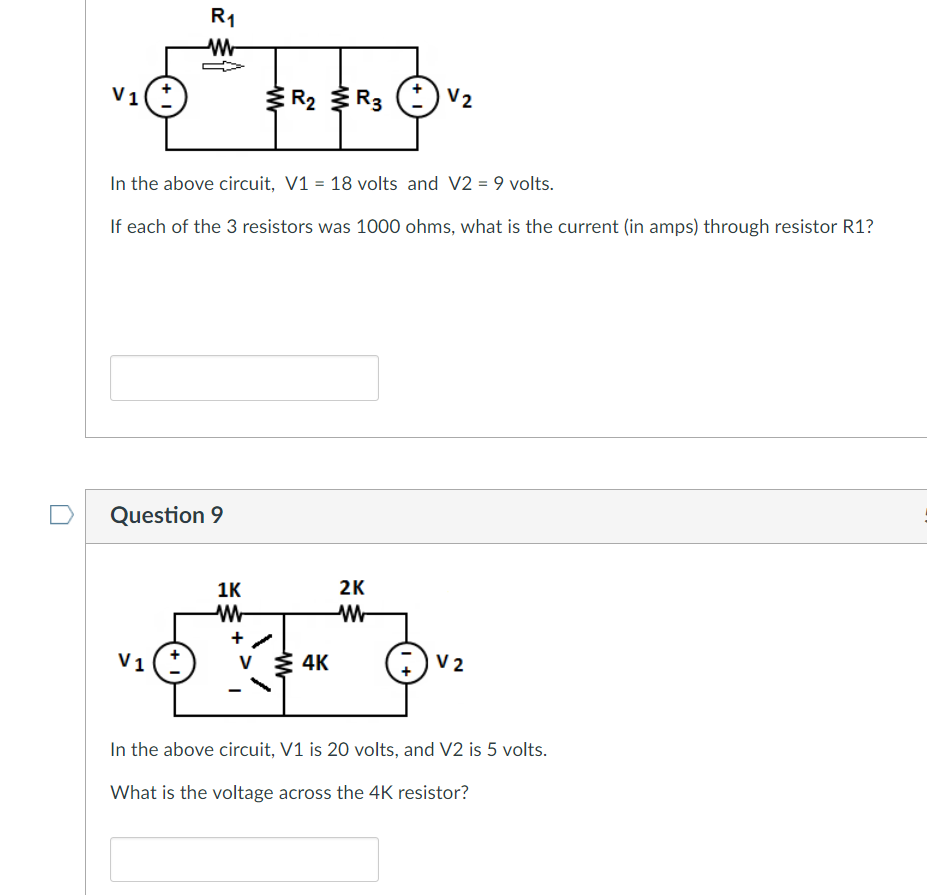R1 2 In the above circuit, V1- 18 volts and V2 -9 volts. If each of the 3 resistors was 1000 ohms, what is the current (in amps) through resistor R1? Question 9 LJK 2K n the above circuit, V1 is 20 volts, and V2 is 5 volts. What is the voltage across the 4K resistor?

• ### R 1 V1 In the above circuit, V1- 15 volts, V2 6 volts, R1 8 ohms...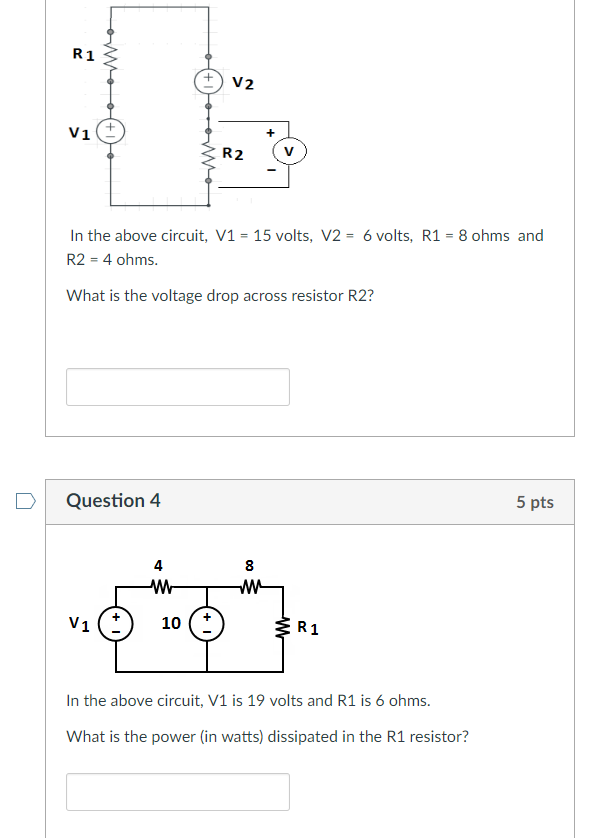R 1 V1 In the above circuit, V1- 15 volts, V2 6 volts, R1 8 ohms and R2 4 ohms. What is the voltage drop across resistor R2? D Question 4 5 pts 4 8 In the above circuit, V1 is 19 volts and R1 is 6 ohms. What is the power (in watts) dissipated in the R1 resistor?

• ### R1 R2 R 3 2 in the above circuit, V1 - 17 volts and v2 -...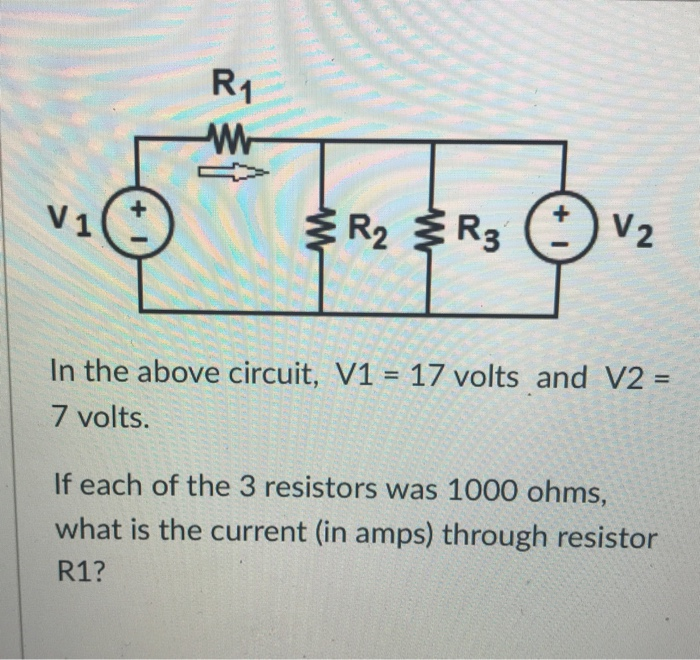R1 R2 R 3 2 in the above circuit, V1 - 17 volts and v2 - 7 volts. If each of the 3 resistors was 1000 ohms, what is the current (in amps) through resistor R1?

• ### R1= 24.8 ohms R2= 13.9 ohms R3= 25.5 ohms V1= 5 volts V2= 11.9 volts V3=...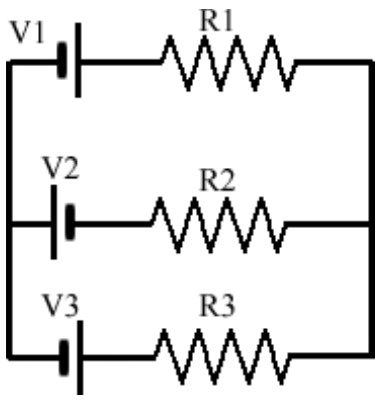R1= 24.8 ohms R2= 13.9 ohms R3= 25.5 ohms V1= 5 volts V2= 11.9 volts V3= 9.1 volts What is the current through the 13.9 ohm resistor? R1 V1 V2 R2 V3 R3

• ### please answer asap V1 = 8 (V) R1 = 8 (12) V2 = 26 (V) Problem...please answer asap V1 = 8 (V) R1 = 8 (12) V2 = 26 (V) Problem 1 Using Kirchhoff's rules in the circuit of image, V4 = 28 (V) V3 = 20 (V) A- Calculate the current in the circuit. B- Show the direction current in the circuit. C- Calculate the voltage across resistor R1 D- Calculate the power delivered to R2 E- After 15 minutes of working of circuit, how much energy is delivered to resistor Rı? R2 =...

• ### What is the voltage V1 (in volts)? What is the voltage V2 (in volts)? What is...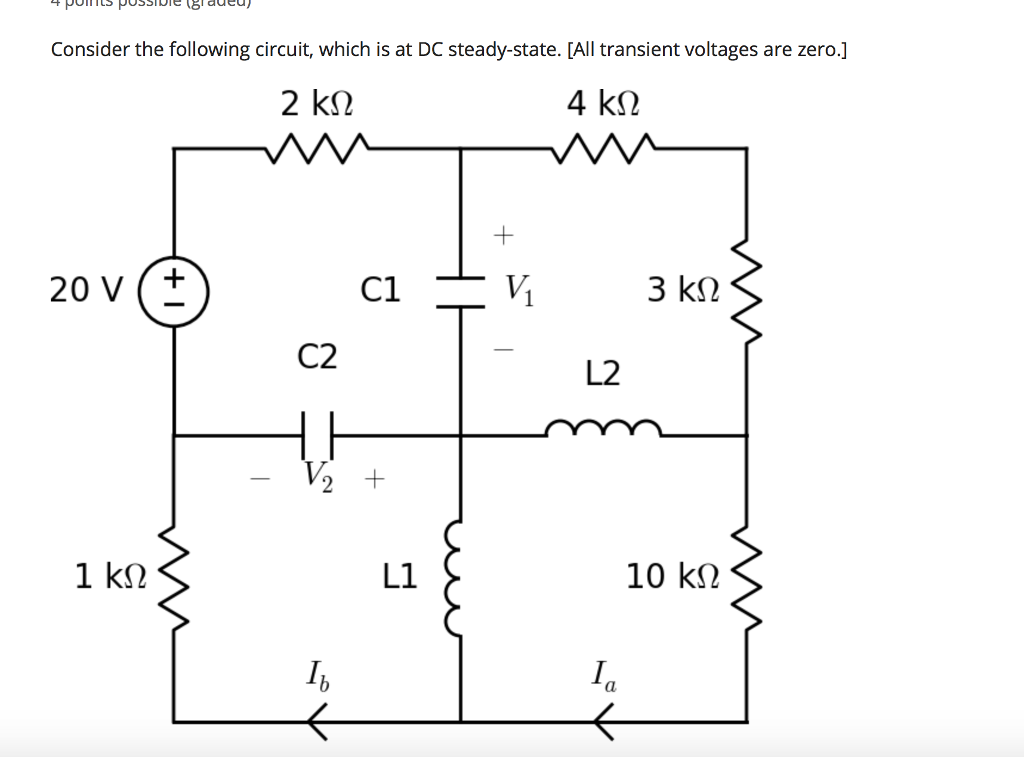What is the voltage V1 (in volts)? What is the voltage V2 (in volts)? What is the current Ia (in mA)? What is the current Ib (in mA)? 8 aded Consider the following circuit, which is at DC steady-state. [All transient voltages are zero.] 3 kS2 C2 L2 L1 10 k? 0

• ### V1 = 8 (V) R1 = 8 (2) V2 = 26 (V) HI Problem 1 Using...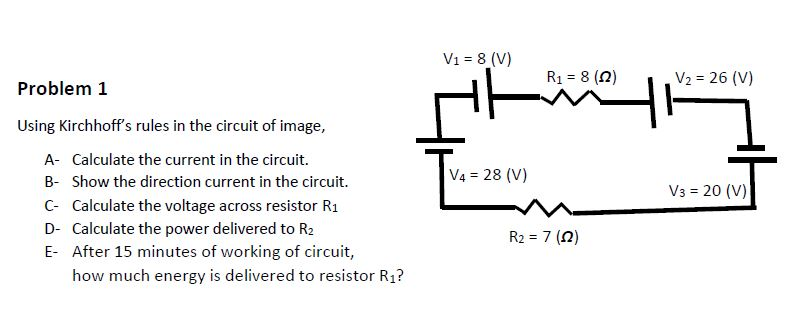V1 = 8 (V) R1 = 8 (2) V2 = 26 (V) HI Problem 1 Using Kirchhoff's rules in the circuit of image, A- Calculate the current in the circuit. B- Show the direction current in the circuit. C- Calculate the voltage across resistor R1 D- Calculate the power delivered to R2 E- After 15 minutes of working of circuit, how much energy is delivered to resistor Rı? V4 = 28 (V) V3 = 20 (V) R2 = 7 (12)

• ### DIODE CIRCUITS: (CONTINUE..) 6c) ID1 ID2 8 V2 V3 3V 6V 1kn "T V1 10V D...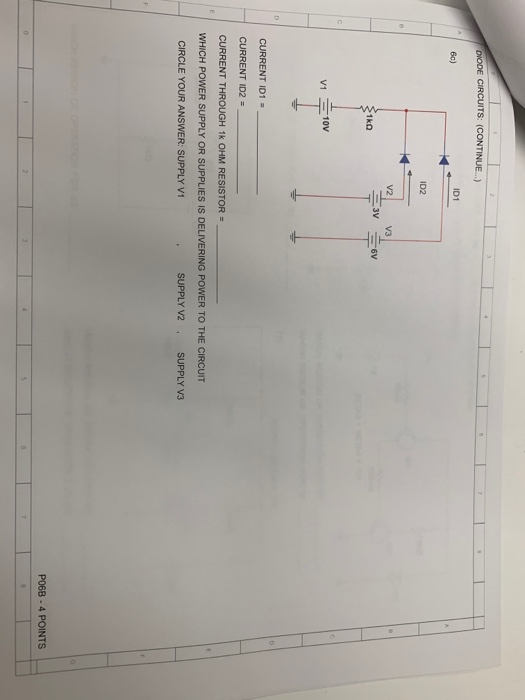DIODE CIRCUITS: (CONTINUE..) 6c) ID1 ID2 8 V2 V3 3V 6V 1kn "T V1 10V D CURRENT ID1 CURRENT ID2 CURRENT THROUGH 1k OHM RESISTOR = WHICH POWER SUPPLY OR SUPPLIES IS DELIVERING POWER TO THE CIRCUIT CIRCLE YOUR ANSWER: SUPPLY V1 SUPPLY V2 SUPPLY V3 P068 4 POINTS

• ### current controlled voltage source 2i R2 V3 EV1 V2 R1 In the circuit above V1 =...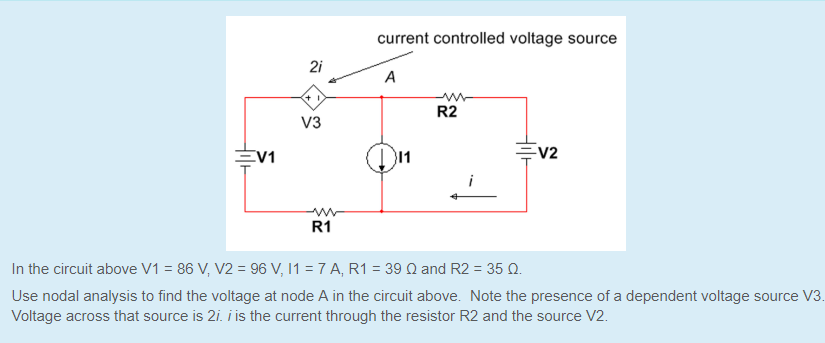current controlled voltage source 2i R2 V3 EV1 V2 R1 In the circuit above V1 = 86V,V2=96 V, 11-7AR1:39 ? and R2 : 35 ?. Use nodal analysis to find the voltage at node A in the circuit above. Note the presence of a dependent voltage source V3 Voltage across that source is 2i. i is the current through the resistor R2 and the source V2

• ### V1 = 8 (V) R1 = 8 (2) V2 = 26 (V) 두 - Problem 1...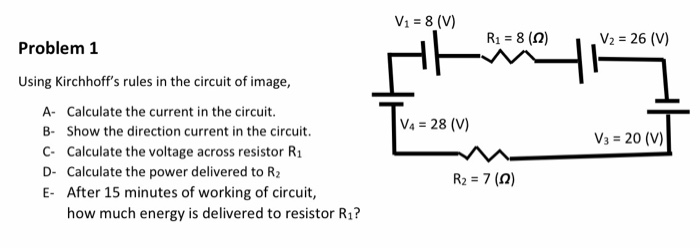V1 = 8 (V) R1 = 8 (2) V2 = 26 (V) 두 - Problem 1 Using Kirchhoff's rules in the circuit of image, A- Calculate the current in the circuit. B- Show the direction current in the circuit. C. Calculate the voltage across resistor R1 D- Calculate the power delivered to R2 E- After 15 minutes of working of circuit, how much energy is delivered to resistor Rı? |VA = 28 (V) Va = 20 (V) R2 = 7...

Free Homework App